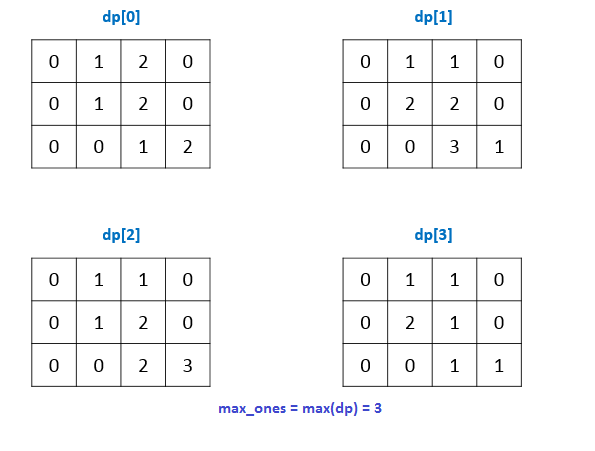## 562. Longest Line of Consecutive One in Matrix

Given a 01 matrix M, find the longest line of consecutive one in the matrix. The line could be horizontal, vertical, diagonal or anti-diagonal.

Example:

```Input:
[[0,1,1,0],
[0,1,1,0],
[0,0,0,1]]
Output: 3
```

Hint: The number of elements in the given matrix will not exceed 10,000.

b'
\n\n

## Solution

\n
\n

#### Approach #1 Brute Force [Accepted]

\n

Algorithm

\n

The brute force approach is really simple. We directly traverse along every valid line in the given matrix: i.e. Horizontal, Vertical, Diagonal aline above and below the middle diagonal, Anti-diagonal line above and below the middle anti-diagonal. Each time during the traversal, we keep on incrementing the if we encounter continuous 1\'s. We reset the for any discontinuity encountered. While doing this, we also keep a track of the maximum found so far.

\n\n

Complexity Analysis

\n
\n
• Time complexity : . We traverse along the entire matrix 4 times.
• \n
• Space complexity : . Constant space is used.
• \n
\n
\n

#### Approach #2 Using 3D Dynamic Programming [Accepted]

\n

Algorithm

\n

Instead of traversing over the same matrix multiple times, we can keep a track of the 1\' along all the lines possible while traversing the matrix once only. In order to do so, we make use of a \$ sized array. Here, , , , are used to store the maximum number of continuous 1\'s found so far along the Horizontal, Vertical, Diagonal and Anti-diagonal lines respectively. e.g. is used to store the number of continuous 1\'s found so far(till we reach the element ), along the horizontal lines only.

\n

Thus, we traverse the matrix in a row-wise fashion only but, keep updating the entries for every appropriately.

\n

The following image shows the filled values for this matrix:

\n
` 0 1 1 0\n\n 0 1 1 0\n\n 0 0 1 1\n`
\n\n

While filling up the , we can keep a track of the length of the longest consecutive line of 1\'s.

\n

Watch this animation for complete process:

\n

!?!../Documents/562_Longest_Line.json:1000,563!?!

\n\n

Complexity Analysis

\n
\n
• \n

Time complexity : . We traverse the entire matrix once only.

\n
• \n
• \n

Space complexity : . array of size is used, where and are the number of rows ans coloumns of the matrix.

\n
• \n
\n
\n

#### Approach #3 Using 2D Dynamic Programming [Accepted]

\n

Algorithm

\n

In the previous approach, we can observe that the current entry is dependent only on the entries of the just previous corresponding row. Thus, instead of maintaining a 2-D matrix for each kind of line of 1\'s possible, we can use a 1-d array for each one of them, and update the corresponding entries in the same row during each row\'s traversal. Taking this into account, the previous 3-D matrix shrinks to a 2-D matrix now. The rest of the procedure remains same as the previous approach.

\n\n

Complexity Analysis

\n
\n
• \n

Time complexity : . The entire matrix is traversed once only.

\n
• \n
• \n

Space complexity : . array of size is used, where is the number of columns of the matrix.

\n
• \n
\n
\n

Analysis written by: @vinod23

\n
'